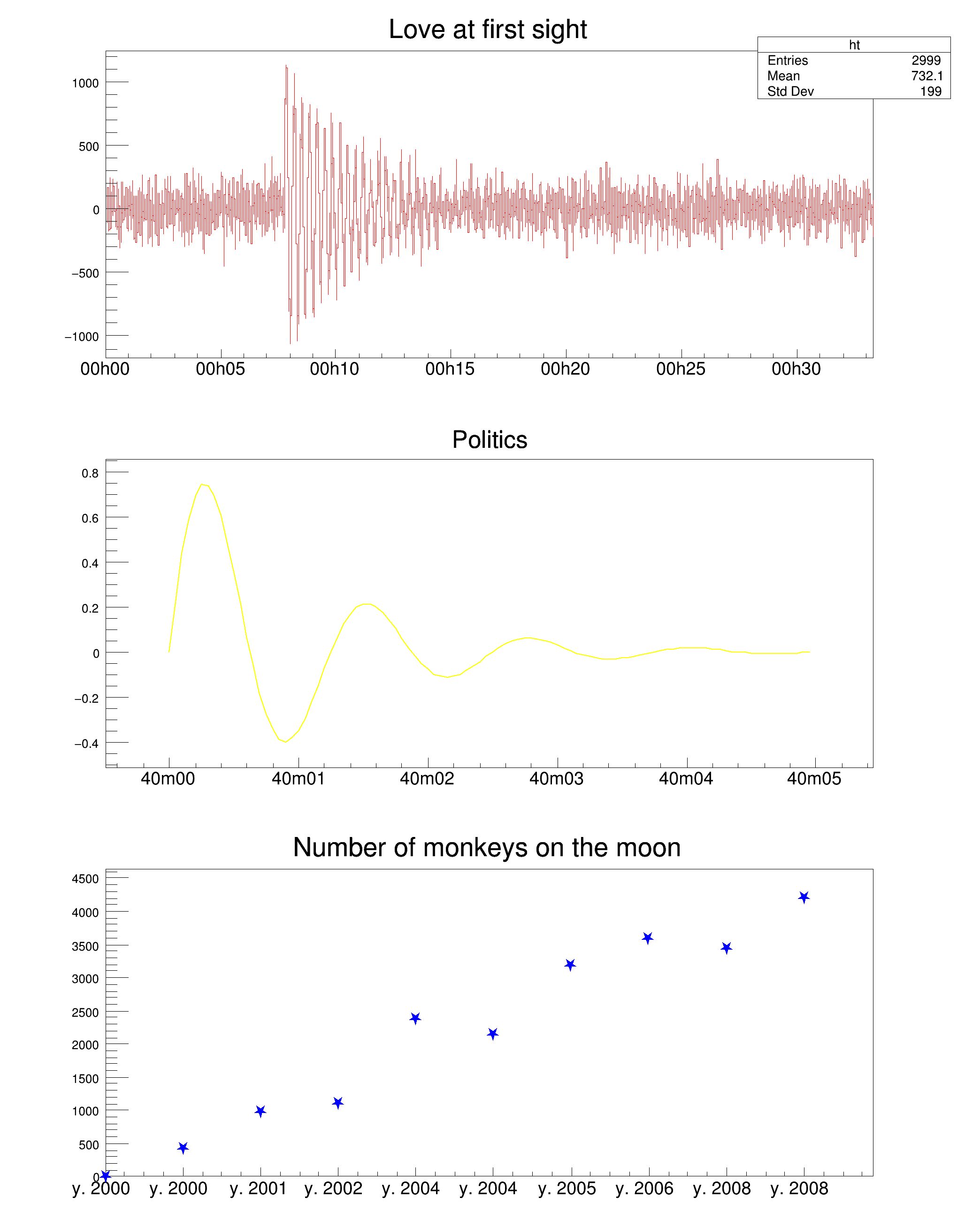ROOT   Reference Guidetimeonaxis.C File Reference

## Detailed DescriptionThis macro illustrates the use of the time mode on the axis with different time intervals and time formats.

Through all this script, the time is expressed in UTC. some information about this format (and others like GPS) may be found at http://tycho.usno.navy.mil/systime.html or http://www.topology.org/sci/time.html

The start time is : almost NOW (the time at which the script is executed) actually, the nearest preceding hour beginning. The time is in general expressed in UTC time with the C time() function This will obviously most of the time not be the time displayed on your watch since it is universal time. See the C time functions for converting this time into more useful structures.#include <time.h>
TCanvas *timeonaxis()
{
time_t script_time;
script_time = time(0);
script_time = 3600*(int)(script_time/3600);
// The time offset is the one that will be used by all graphs.
// If one changes it, it will be changed even on the graphs already defined
gStyle->SetTimeOffset(script_time);
TCanvas *ct = new TCanvas("ct","Time on axis",10,10,700,900);
ct->Divide(1,3);
int i;
//### Build a signal : noisy damped sine
// Time interval : 30 minutes
gStyle->SetTitleH(0.08);
float noise;
TH1F *ht = new TH1F("ht","Love at first sight",3000,0.,2000.);
for (i=1;i<3000;i++) {
noise = gRandom->Gaus(0,120);
if (i>700) {
noise += 1000*sin((i-700)*6.28/30)*exp((double)(700-i)/300);
}
ht->SetBinContent(i,noise);
}
ct->cd(1);
ht->SetLineColor(2);
ht->GetXaxis()->SetLabelSize(0.05);
ht->Draw();
// Sets time on the X axis
// The time used is the one set as time offset added to the value
// of the axis. This is converted into day/month/year hour:min:sec and
// a reasonable tick interval value is chosen.
//### Build a simple graph beginning at a different time
// Time interval : 5 seconds
float x, t;
for (i=0;i<100;i++) {
x[i] = sin(i*4*3.1415926/50)*exp(-(double)i/20);
t[i] = 6000+(double)i/20;
}
TGraph *gt = new TGraph(100,t,x);
gt->SetTitle("Politics");
ct->cd(2);
gt->SetLineColor(5);
gt->SetLineWidth(2);
gt->Draw("AL");
gt->GetXaxis()->SetLabelSize(0.05);
// Sets time on the X axis
//### Build a second simple graph for a very long time interval
// Time interval : a few years
float x2, t2;
for (i=0;i<10;i++) {
x2[i] = gRandom->Gaus(500,100)*i;
t2[i] = i*365*86400;
}
TGraph *gt2 = new TGraph(10,t2,x2);
gt2->SetTitle("Number of monkeys on the moon");
ct->cd(3);
gt2->SetMarkerColor(4);
gt2->SetMarkerStyle(29);
gt2->SetMarkerSize(1.3);
gt2->Draw("AP");
gt2->GetXaxis()->SetLabelSize(0.05);
// Sets time on the X axis
//
// One can choose a different time format than the one chosen by default
// The time format is the same as the one of the C strftime() function
// It's a string containing the following formats :
//
// for date :
// %a abbreviated weekday name
// %b abbreviated month name
// %d day of the month (01-31)
// %m month (01-12)
// %y year without century
// %Y year with century
//
// for time :
// %H hour (24-hour clock)
// %I hour (12-hour clock)
// %p local equivalent of AM or PM
// %M minute (00-59)
// %S seconds (00-61)
// %% %
// The other characters are output as is.
gt2->GetXaxis()->SetTimeFormat("y. %Y %F2000-01-01 00:00:00");
return ct;
}
double
Definition: Converters.cxx:921
static const double x2
double sin(double)
double exp(double)
R__EXTERN TRandom * gRandom
Definition: TRandom.h:62
R__EXTERN TStyle * gStyle
Definition: TStyle.h:412
virtual void SetLabelSize(Float_t size=0.04)
Set size of axis labels.
Definition: TAttAxis.cxx:203
virtual void SetLineWidth(Width_t lwidth)
Set the line width.
Definition: TAttLine.h:43
virtual void SetLineColor(Color_t lcolor)
Set the line color.
Definition: TAttLine.h:40
virtual void SetMarkerColor(Color_t mcolor=1)
Set the marker color.
Definition: TAttMarker.h:38
virtual void SetMarkerStyle(Style_t mstyle=1)
Set the marker style.
Definition: TAttMarker.h:40
virtual void SetMarkerSize(Size_t msize=1)
Set the marker size.
Definition: TAttMarker.h:41
virtual void SetTimeDisplay(Int_t value)
Definition: TAxis.h:161
virtual void SetTimeFormat(const char *format="")
Change the format used for time plotting.
Definition: TAxis.cxx:1020
The Canvas class.
Definition: TCanvas.h:23
Definition: TCanvas.cxx:708
A TGraph is an object made of two arrays X and Y with npoints each.
Definition: TGraph.h:41
virtual void SetTitle(const char *title="")
Change (i.e.
Definition: TGraph.cxx:2339
virtual void Draw(Option_t *chopt="")
Draw this graph with its current attributes.
Definition: TGraph.cxx:769
TAxis * GetXaxis() const
Get x axis of the graph.
Definition: TGraph.cxx:1640
1-D histogram with a float per channel (see TH1 documentation)}
Definition: TH1.h:575
TAxis * GetXaxis()
Definition: TH1.h:320
virtual void SetBinContent(Int_t bin, Double_t content)
Set bin content see convention for numbering bins in TH1::GetBin In case the bin number is greater th...
Definition: TH1.cxx:9062
virtual void Draw(Option_t *option="")
Draw this histogram with options.
Definition: TH1.cxx:3073
void Divide(Int_t nx=1, Int_t ny=1, Float_t xmargin=0.01, Float_t ymargin=0.01, Int_t color=0) override